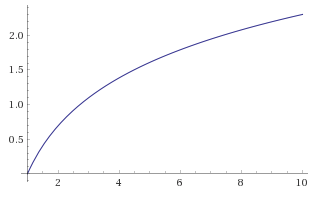Some things make your brain shut off. You just see complex, not looking closer. Big O Notation can be like that. It’s a way to describe the efficiency of an algorithm, how the “speed” is affected by the growth of the input, `n`. Computing it can be tricky, so start off just learning how to read it.

Here are some simple examples.

## O(1)

``````const funk = (n) => { }
``````

Constant - It doesn’t matter what the size of `n` is. This function will always take the same amount of time to run.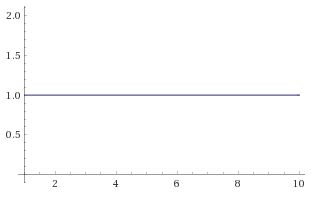## O(n)

``````const funk = (n) => {
n.forEach(x => { })
}
``````

Linear - This as the size of `n` grows, the time to run this function will grow linearly.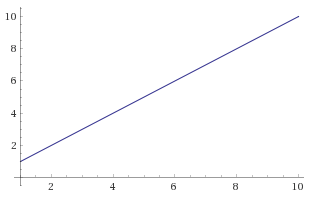## O(n2)

``````const funk = (n) => {
n.forEach(x => {
n.forEach(y => { })
})
}
``````

Squared - For each item in `n` we loop, then again within each loop.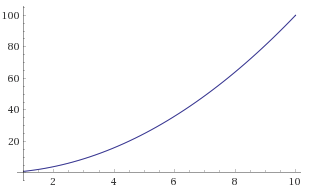## O(2n)

``````const funk = (n) => {
return (n <= 1)
? n
: funk(n - 2) + funk(n - 1)
}
``````

Exponential - The time doubles for each increase of `n`; it grows exponentially. This is OK for small inputs, but will quickly start to suck as `n` grows.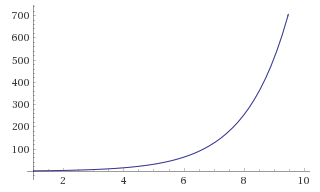## O(log n)

``````const funk = (n) => {
return (n <= 1)
? n
: funk(n / 2)
}
``````

Logarithmic - The time for this function grows more slowly as `n` increases, logarithmically, the inverse of exponential growth. These kind of algorithms cost a lot initially, but pay off for big inputs.